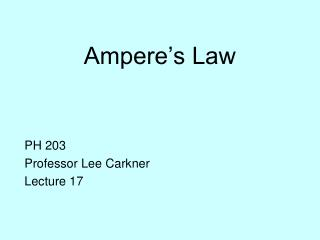DownloadDownload PresentationAmpere’s Law

Ampere’s Law

Télécharger la présentationAmpere’s Law

- - - - - - - - - - - - - - - - - - - - - - - - - - - E N D - - - - - - - - - - - - - - - - - - - - - - - - - - -
Presentation Transcript

1. Ampere’s Law PH 203 Professor Lee Carkner Lecture 17

2. Test 2 on Monday • Covers everything since last test through Wednesday • 10 multiple choice (20 points) • 4 problems (20 points each) • Equations and constants given • but not labeled • Bring calculator • No PDA’s, no cellphones, no sharing • Study • PAL’s • Notes • Homework

3. Currents and Magnetism • It is also true that moving charged particles produce magnetic fields • Serious magnetic fields are produced by currents • What is the magnitude and direction of these fields?

4. Magnetic Field from a Current in a Wire • Needle deflected tangentially to the wire cross section • How can we find the direction and magnitude of the B field for any situation?

5. Grasp the wire with your thumb in the direction of the current and your curled fingers indicate the direction of the field Right Hand Rule Revisited

6. Ampere’s Law • To find the magnitude of the B field we use Ampere’s law • The integral of the product of ds and B around the entire path is equal to m0i • Where m0 = 4p X 10-7 T m /A and is called the permeability of free space ∫ B ds = m0i • where i is the charge enclosed by the path

7. B Field for a Wire ∫ B ds = m0i or B ∫ ds = m0i • Since B is the same everywhere around the circle B 2pr = m0i B = m0i/2pr • Magnetic field a distance r from a long straight wire with current i

8. B Field within a Wire • e.g. within the wire • In this case the B field is still B = m0ienc/2pr • If the total radius of the wire is R ienc = i(pr2/pR2) B = (m0ir)/(2pR2)

9. Force on Two Parallel Wires • The B field then will exert a force on the other wire B = m0i/2pd F = BiL = m0iiL/2pd F = (m0i1i2L)/(2pd)

10. Next Time • Read 29.5-29.6 • Problems: Ch 29, P: 9, 28, 37, 42, 49

11. Consider a charged particle in a circular orbit in a magnetic field. If the charge on the particle is doubled, and the velocity of the particle is doubled, what happens to the radius of the orbit? ¼ the original ½ the original the radius stays the same 2 times the original 4 times the original

12. The force on a current-carrying wire in a magnetic field is strongest when, • the current is parallel to the field lines • the current is at a 30 degree angle to the field lines • the current is at a 45 degree angle to the field lines • the current is at a 60 degree angle to the field lines • the current is perpendicular to the field lines

13. Consider a vector that stands straight out from the face of a loop of wire that carries a current. The magnetic torque on the loop will be greatest when, • the vector is aligned with the magnetic field • the vector is at a 30 degree angle to the magnetic field • the vector is at a 45 degree angle to the magnetic field • the vector is at a 60 degree angle to the magnetic field • the vector is perpendicular to the magnetic field